Maths-
General
Easy

Question

# 45.76 + 36.69

## The correct answer is: 82.45

### The addition of the decimal numbers is done as follows.Step1: Starting from the right.While adding two number which are more than two digit, we start adding them from the right side.Here in this question we are given decimal numbers which are as follows.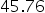and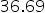.First we will add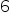and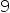which gives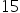and fromwe will give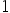carry to next two number when we add.The next two numbers we will add are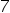andwhich gives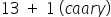. Now from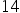we will again givecarry to next two numberStep2: Adding after decimalNow we will add numbers that are after decimalsWe will addand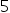which gives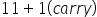. Now from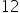we will take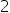and givecarry to next two numberAt final we will add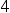and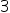which gives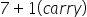.So, the result of addition is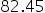#### With Turito Foundation.#### Get an Expert Advice From Turito.